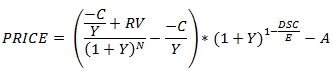# SQL Server pricing function for regular bonds

RPI

Updated: 31 May 2014

Use RPI to calculate the price or yield for a bond that pays periodic interest and has a par value of 100. The formula for price with more than one coupon period to redemption is:Where
C = 100 * coupon rate / frequency
Y = yield / frequency
RV = redemption value
DSC = number of days from settlement to coupon
N = the number of coupons between the settlement date and the maturity date
E = the number of days in the current coupon period
A = C * accrued days / E
When the next coupon is paid at maturity the formula for price is:Where
C = 100 * coupon rate / frequency
Y = yield / frequency
RV = redemption value
DSR = number of days from settlement to redemption
E = the number of days in the current coupon period
A = C * accrued days / E
The RPI function allows you to pass values for A, DSC/DSR, E, RV, and N directly into the equation and automatically calculates Y and C.
Syntax
SELECT [wctFinancial].[wct].[RPI](
<@A, float,>
,<@DSC, float,>
,<@E, float,>
,<@N, float,>
,<@R, float,>
,<@Y, float,>
,<@P, float,>
,<@F, int,>
,<@RV, float,>)
Arguments
@A
the accrued days. @A is an expression of type float or of a type that can be implicitly converted to float.
@DSC
the days from settlement to next coupon date (when @N > 1) or the days from settlement to redemption (when @N = 1). @DSC is an expression of type float or of a type that can be implicitly converted to float.
@E
the number of days in the settlement period. @E is an expression of type float or of a type that can be implicitly converted to float.
@N
the number of coupons between settlement and maturity. @Yld is an expression of type float or of a type that can be implicitly converted to float.
@R
the annual coupon rate. @R is an expression of type float or of a type that can be implicitly converted to float.
@Y
the yield on the bond. @Y is an expression of type float or of a type that can be implicitly converted to float.
@P
the price of the bond. @P is an expression of type float or of a type that can be implicitly converted to float.
@F
the number of coupon payments per year. For annual payments, @F = 1; for semi-annual, @F= 2; for quarterly, @F= 4; for bi-monthly, @F = 6; for monthly @F = 12. @F is an expression of type int or of a type that can be implicitly converted to int.
@RV
the redemption value of the security. @RV is an expression of type float or of a type that be implicitly converted to float.
Return Type
float
Remarks
·         If @A is NULL then @A = 0.
·         If @E is NULL then @E =180.
·         If @DSC is NULL then @DSC = @E - @A.
·         If @N is NULL then @N = 2.
·         If @R is NULL then @R = 0.
·         If @F is NULL then @F = 2.
·         If @RV is NULL then @RV = 100.
·         If @Y is NULL and @P is NULL then NULL is returned.
·         If @E = 0 then NULL is returned.
·         If @F = 0 then NULL is returned.
·         C = 100 * @R/@F
·         Y = @Y/@F
·         If @Y is not NULL then the function calculates the price from the inputs otherwise the function calculates the yield.
Examples
In this example we calculate the price for a bond with an annual coupon rate of 2.50% paid twice a year with a redemption value of 100. There are 137 accrued days, 45 days until the next coupon, 182 days in the coupon period and 41 coupons remaining. The yield on the bond is 2.76%
SELECT
wct.RPI(
137,     --@A
45,      --@DSC
182,     --@E
41,      --@N
.025,    --@R
0.0276,  --@Y
NULL,    --@P
2,       --@F
100      --@RV
) as PRICE

This produces the following result
PRICE
----------------------
96.0043799057024

In this example, we calculate the price of a zero-coupon bond with 137 accrued days, 45 days to the next coupon date, 182 days in the settlement period, and 61 coupon periods until maturity. The yield on the bond is 3.01%
SELECT
wct.RPI(
137,     --@A
45,      --@DSC
182,     --@E
61,      --@N
0.0,     --@R
0.0301,  --@Y
NULL,    --@P
2,       --@F
100      --@RV
) as PRICE

This produces the following result.
PRICE
----------------------
40.6583576113141

In this example we calculate the price of a bond settling in the final coupon period.
SELECT
wct.RPI(
106,     --@A
74,      --@DSC
180,     --@E
1,       --@N
0.019,   --@R
0.0005,  --@Y
NULL,    --@P
2,       --@F
100      --@RV
) as PRICE

This produces the following result.
PRICE
----------------------
100.380181205142

Here we calculate the yield of a bond.
SELECT
wct.RPI(
32,         --@A
152,        --@DSC
184,        --@E
41,         --@N
0.0257,     --@R
NULL,       --@Y
98.123291--@P
2,          --@F
100         --@RV
) as YIELD

This produces the following result.
YIELD
----------------------
0.0268999998648661

Here's an example of the yield calculation returning a negative yield.
SELECT
wct.RPI(
31,         --@A
149,        --@DSC
180,        --@E
1,          --@N
0.0257,     --@R
NULL,       --@Y
101,        --@P
2,          --@F
98          --@RV
) as YIELD

This produces the following result.
YIELD
----------------------
-0.0462187493233163

### SupportCopyright 2008-2022 Westclintech LLC         Privacy Policy        Terms of Service